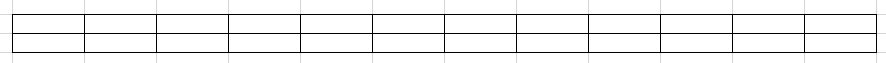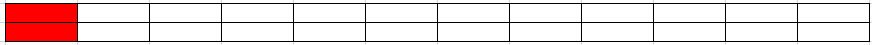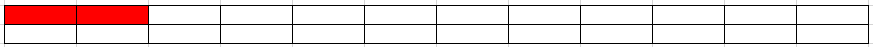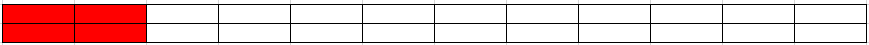# 递归算法--斐波那契数列

## 大家都知道斐波那契数列，现在要求输入一个整数n，请你输出斐波那契数列的第n项（从0开始，第0项为0）。n<=39

public class Solution {
public int Fibonacci(int n) {
if(n == 0)
return 0;
if(n == 1)
return 1;
return Fibonacci(n-2) + Fibonacci(n-1);
}
}

public class Solution {
public int Fibonacci(int n) {
if(n < 2)
return n;
int f = 0, g = 1;
int result = 0;
for(int i = 1; i < n; i++){
result = f + g;
f = g;
g = result;
}
return result;
}
}

### 分析1. 第一步这样覆盖2. 第一步这么覆盖public class Solution {
public int RectCover(int target) {
if(target  <= 1){
return 1;
}
if(target*2 == 2){
return 1;
}else if(target*2 == 4){
return 2;
}else{
return RectCover((target-1))+RectCover(target-2);
}
}
}